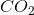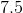# SAT II Chemistry : Balancing Equations

## Example Questions

### Example Question #1 : Stoichiometry

When the equation for the reaction shown below is balanced with the lowest whole-number coefficients, what is the sum of the coefficients of the reactants?

___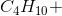___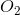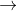___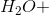___